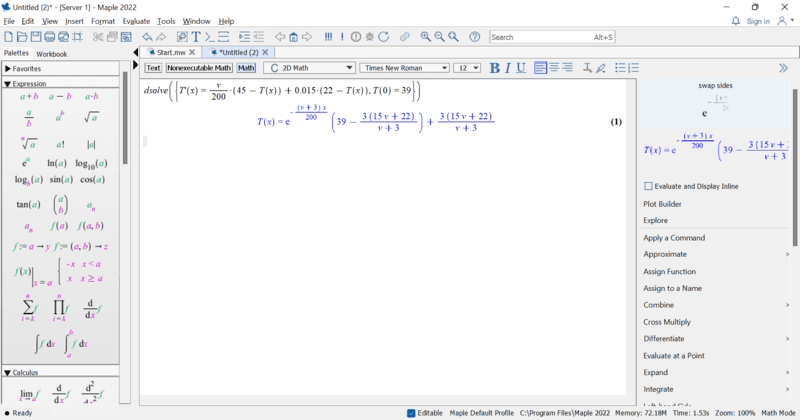# Finding the maximum size of an Initial Value Problem coefficient

• MathMan2022
In summary, the given conversation discusses finding the maximum size of the coefficient v in the IVP equation for temperature T in a tub filled with water. The equation is diff(T(x), x) = v/200*(45 - T(x)) + 0.015*(22 - T(x)) where T(0)=39. The problem is to calculate the maximum value of v for a person taking a 40-minute bath, with the condition that the water in the tub should not fall below 37 degrees Celsius. The screenshot shows a solution using Maple's dsolve function, but the method for finding the maximum value of v is unclear.

#### MathMan2022

Homework Statement
find the maximum size of a IVP coefficient size?
Relevant Equations
diff(T(x), x) = v/200*(45 - T(x)) + 0.015*(22 - T(x)) where T(0)=39
The following IVP
diff(T(x), x) = v/200*(45 - T(x)) + 0.015*(22 - T(x)) where T(0)=39

Describes the tempetatur T in celcius at the time x of a tub filled with water. A tub which is filled with hot water at rate of v l/min.

Lets say I am told that a guy takes a 40 min bath, and during those 40 min he doesn't want the water in the tub to become colder than 37 c.
How would I go about calculating the maximum size of v for that periode?

MathMan2022 said:
Homework Statement:: find the maximum size of a IVP coefficient size?
Relevant Equations:: diff(T(x), x) = v/200*(45 - T(x)) + 0.015*(22 - T(x)) where T(0)=39

The following IVP
diff(T(x), x) = v/200*(45 - T(x)) + 0.015*(22 - T(x)) where T(0)=39

Describes the tempetatur T in celcius at the time x of a tub filled with water. A tub which is filled with hot water at rate of v l/min.

Lets say I am told that a guy takes a 40 min bath, and during those 40 min he doesn't want the water in the tub to become colder than 37 c.
How would I go about calculating the maximum size of v for that periode?

Welcome to PF.